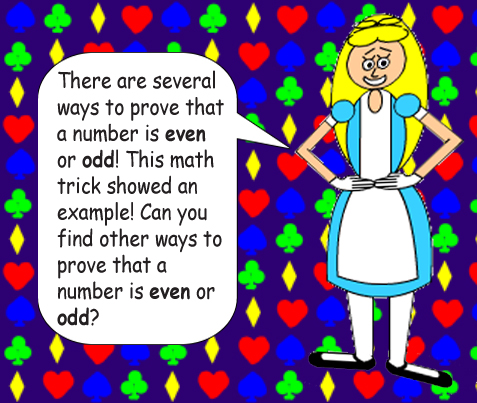## Observe the formula in T.V. Man's screen!## Examples:Y cannot be equal to zero(0) because division by zero is undefined. And according to that well-known exponential rule:### Note: If Y is equal to 1, then the radical sign is not necessary!

If X is negative & Y is even, then you will get an imaginary number, which will then be squared to a negative real number!However, if X is negative but Y is odd, then you'll simply get a positive real number.